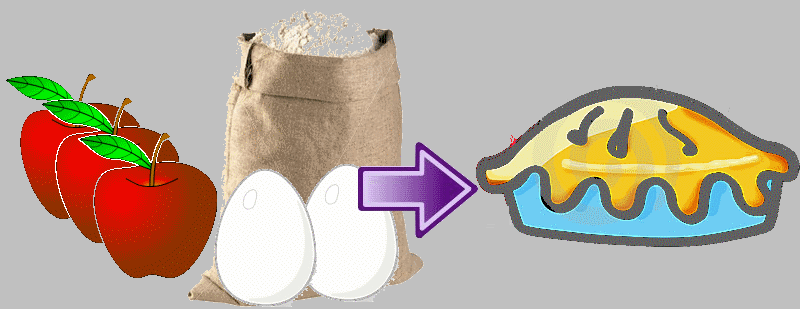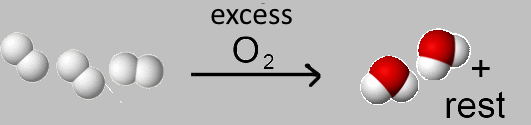Search:

# The yield of a chemical reaction

## Example 1

A diesel engine provides only 45 % of the power which it could provide if the whole energy liberated by the combustion was transformed to usable energy. The losses have multiple causes which are mostly anavoidable.## Example 2To make an apple cake , you need 3 apples, 2 eggs and enough flour.Unfortunately this time three apples were rotten and with your 12 apples you can make only 3 cakes, while you had planned for 4 ! The yield with respect to the apples is $\rho$ $=$ $\frac{3}{4}$ $=$ $0.75$ or $\rho$ $=$ $75\%$ (from the $100\%$ that you should have made).Unfortunately this time 3 eggs were broken and with 9 eggs you realise only 3 cakes, while you had planned for $\frac{9}{2}$ ! ! The yield with respect to the eggs is $\rho$ $=$ $\frac{3}{\frac{9}{2}}=0.666$ or $\rho$ $=$ $66.6\%$ (from the $100\%$ that you should have made).

## Definition of yield in chemistry

The yield of a chemical reaction is the ration of the number of moles of a product obtained actually by the number of moles of this producttheoretically obtainable. $\rho=\frac{n_{actually\; obtained}}{n_{theoretically\; obtainable}}$ The yield is often given in %, then $\rho$ is multiplied by 100: $\rho_{\%}$ $=$ $\frac{n_{actually\; obtained}\cdot 100}{n_{theoretically\; obtainable}}$

## Example3 molecules $H_2$ should give 3 molecules $H_2O$ but actually they produce only 2 (because of losses while water is collected, lack of watertight integrity of the containers, etc...) The yield with respect to hydrogen is therefore $\rho$ $=$ $\frac{2}{3}$ $=$ $0.666$ or $\rho$ $=$ $66.6\%$

.